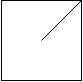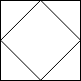959. Regions Cut By Slashes
Medium
2.7K
513

An `n x n` grid is composed of `1 x 1` squares where each `1 x 1` square consists of a `'/'`, `'\'`, or blank space `' '`. These characters divide the square into contiguous regions.

Given the grid `grid` represented as a string array, return the number of regions.

Note that backslash characters are escaped, so a `'\'` is represented as `'\\'`.

Example 1:```Input: grid = [" /","/ "]
Output: 2
```

Example 2:```Input: grid = [" /","  "]
Output: 1
```

Example 3:```Input: grid = ["/\\","\\/"]
Output: 5
Explanation: Recall that because \ characters are escaped, "\\/" refers to \/, and "/\\" refers to /\.
```

Constraints:

• `n == grid.length == grid[i].length`
• `1 <= n <= 30`
• `grid[i][j]` is either `'/'`, `'\'`, or `' '`.
Accepted
45K
Submissions
65.1K
Acceptance Rate
69.1%

Seen this question in a real interview before?
1/4
Yes
No

Discussion (0)

Related Topics